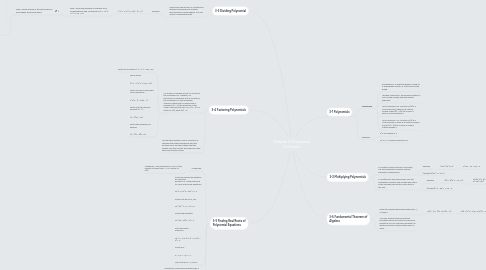# Chapter 3 Polynomial Functions

Get Started. It's FreeChapter 3 Polynomial Functions## 1. 3-1 Polynomials

### 1.1. Vocabulary

1.1.1. End Behavior- is what the graph is doing as it approaches infinity, or at the ends of the graph.

1.1.2. Leading Coefficient- the numbers written in front of the variable with the largest exponent.

1.1.3. Local Maximum-For function f,f(a) is a local maximum if there is an interval around a that f(x) < f(a) for a every x value in interval except a.

1.1.4. Local Minimum- For function f,f(a) is a local minimum if there is an interval around a that f(x) > f(a) for a every x value in interval except a.

### 1.2. Examples

1.2.1. x^3 The degree is 3

1.2.2. 2x^2+x-1 Leading coeficient is 2

## 2. 3-2 Multiplying Polynomials

### 2.1. To multiply a polynomial by a monomial, use the Distributive Property and the Properties of Exponents.

2.1.1. Example

2.1.1.1. 1.)4y^2(y^2+3)

2.1.1.1.1. 2.)4y2 ∙ y2 + 4y2 ∙ 3

### 2.2. To multiply any two polynomials, use the Distributive Property and multiply each term in the second polynomial by each term in the first.

2.2.1. Example

2.2.1.1. 1.)(a – 3)(a^2 – 5a + 2)

2.2.1.1.1. 2.)a(a^2) + a(–5a) + a(2) – 3(a^2) – 3(–5a) –3(2)

2.2.2. (Answer)a^3 – 8a^2 + 17a – 6

## 3. 3-3 Dividing Polynomial

### 3.1. Polynomial long division is a method for dividing a polynomial by another polynomialsof a lower degree. It is very similar to dividingnumbers.

3.1.1. Example

3.1.1.1. (–y^2 + 2y^3 + 25) ÷ (y – 3)

3.1.1.1.1. Step 1 Write the dividend in standard form, includingterms with a coefficient of 0. 2y^3 – y^2 + 0y + 25

## 4. 3-4 Factoring Polynomials

### 4.1. If a number is divided by any of its factors, the remainder is 0. Likewise, if a polynomial is divided by any of its factors, the remainder is 0.The Remainder Theorem states that if a polynomial is divided by (x – a), the remainder is the value of the function at a. So, if (x – a) is a factor of P(x), then P(a) = 0.

4.1.1. Factor by Grouping: x^3 – x^2 – 25x + 25.

4.1.2. Group terms. (x^3 – x^2) + (–25x + 25) Factor common monomials from each group. x^2(x – 1) – 25(x – 1) Factor out the common binomial (x – 1). (x – 1)(x2 – 25) Factor the difference of squares. (x – 1)(x – 5)(x + 5)

## 5. 3-5 Finding Real Roots of Polynomial Equations

### 5.1. Vocabulary

5.1.1. Multiplicity- The multiplicity of root r is the number of times that x – r is a factor of P(x).-

## 6. 3-6 Fundamental Theorem of Algebra

### 6.1. Write the simplest polynomial with roots –1, 2/3,and 4.

6.1.1. P(x) = (x + 1)(x –2/3 )(x – 4)

6.1.1.1. P(x) = (x^2+ 1/3x –2/3 )(x – 4)

6.1.1.1.1. P(x) = x^3 – 11/3x^2 – 2x +8/3